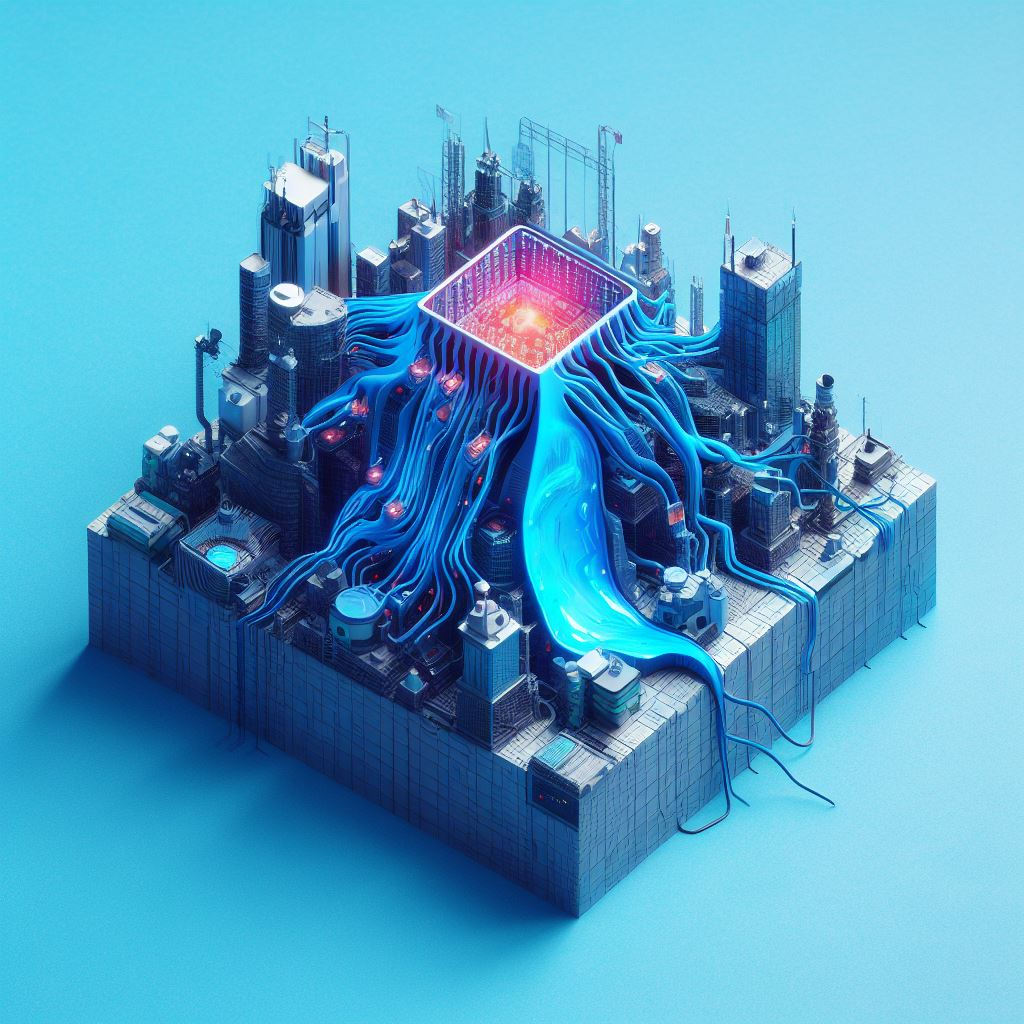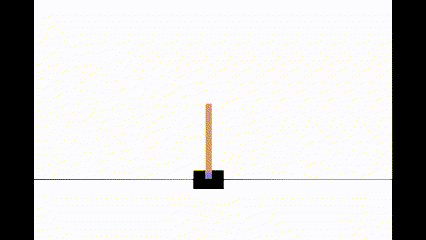## Deep Q Learning with PyTorch

29 Oct 2023# Introduction

This blog is going to be my second one on Reinforcement Learning. You can check out the first one here. This blog will show how to use Deep Q Learning (DQN) to solve a reinforcement learning task. As an example, we will deploy DQN to solve the classic CartPole control task.

Let’s start with some basics before we get into the code. DQN is a powerful technique that uses a neural network instead of a q-table to estimate the q-values for a given state and decide on what action to take in that state. This makes it useful for continuous tasks because unlike a discrete state space, we cannot effectively create a q-table. We would have to resort to either a tile coding or a state aggregation approach. But this may result in a very high dimensionality q-table for many control tasks. This is where DQN comes in.

This blog will use PyTorch to create and train the deep neural network. We get the cartpole environment from the OpenAI Gym package. The aim of our RL agent in the CartPole environment is to balance the pole by moving the cart left or right.

# General Workflow

This is the general procedure to implement DQN for an RL control task.

1. Create an environment
2. Describe the Deep Neural Network Model
3. Create a Replay Memory to store the experiences of the agent.
4. Create a Policy
5. Training and Exploration
6. Save the DQN model for future use.

You can see that the overall workflow is not very complex. That’s the power of using DQN. You can solve very difficult tasks in just a few lines of code, and PyTorch makes it even faster.

# Setting up the project

Like always, let’s begin with setting up the project.

1. Setup a Python virtual environment using this command

For Windows:

python -m venv venv
venv\\Scripts\\activate

For Linux/Mac:

python -m venv venv
source venv/bin/activate 
1. Install the dependencies
pip install torch gymnasium 
1. Create a new cartpole.ipynb file and import the following packages
import copy

import torch
from torch import nn
from torch.functional import F

import gymnasium

from tqdm import tqdm


# The Environment

Using gym we can create our cartpole environment. The main methods we will be using from the environment are the reset and step methods. But these methods give an output that is either just a scalar (reward), vector (state), or Boolean (terminated, truncated). PyTorch cannot directly use these outputs because PyTorch expects tensors as inputs. Thus, we must override these environment methods to return the outputs as tensors. This is how we do it. We create a wrapper around the gym environment using gym.Wrapper class.

class PreProcessEnv(gym.Wrapper):

def __init__(self, env):
gym.Wrapper.__init__(self, env)

def step(self, action):
action = action.item()
obs, reward, terminated, truncated, info = self.env.step(action)

obs = torch.from_numpy(obs).unsqueeze(0).float()
reward = torch.tensor(reward).view(-1, 1)
terminated = torch.tensor(terminated).view(-1, 1)
truncated = torch.tensor(truncated).view(-1, 1)

return obs, reward, terminated, truncated, info

def reset(self):
obs = self.env.reset()
obs = torch.from_numpy(obs).unsqueeze(0).float()
return obs

This code essentially converts the obs, reward, terminated, truncated, info variables to tensors and adds another dimension. We will see why we do this in a moment, but we are essentially adding a batch dimension.

With the wrapper in place, now we can create the environment.

env = gym.make("CartPole-v1")
env = PreProcessEnv(env)

This environment has 4 states :

• Cart Position
• Cart Velocity
• Pole Angle
• Pole Angular Velocity.

The action space is comprised of 2 actions :

• 0: Push cart to the left
• 1: Push cart to the right

The episode terminates when the pole crosses a certain pole angle (12 degrees) or if the cart moves out of the screen. The episode is truncated when the agent can balance the pole for 500 frames.

# The Deep Neural Network

Next, let us create a deep neural network to predict the q values for the input state.

class DQNetworkModel(nn.Module):
def __init__(self, in_channels, out_classes):
super().__init__()
self.layers = nn.Sequential(
nn.Flatten(),
nn.Linear(in_channels, 128),
nn.ReLU(),
nn.Linear(128, 64),
nn.ReLU(),
nn.Linear(64, out_classes),
)

def forward(self, x):
return self.layers(x)

As you can guess, this is just a simple, fully connected neural network with two hidden layers. This will take our state, a vector of size 4, as input and give as output the q-values corresponding to each action (2 actions in this case).

Let’s initiate our model:

q_network = DQNetworkModel(env.observation_space.shape, env.action_space.n).to(device)

target_q_network = copy.deepcopy(q_network).to(device).eval()

As you can see, we have initiated two models. The first model is the one that is trained. The second model is used to calculate the target for our training loss function. We will see more about this in the training loop.

# The Replay Memory

The replay memory is used to save the experiences that our agent accumulates. We then sample from these experiences to train the model. We will implement the replay memory as a Python class with a fixed capacity and a list of memories.

class ReplayMemory:
def __init__(self, capacity=100000):
self.capacity = capacity
self.memory = []
self.position = 0

def insert(self, transition):
if len(self.memory) < self.capacity:
self.memory.append(None)
self.memory[self.position] = transition
self.position = (self.position + 1) % self.capacity

def can_sample(self, batch_size):
return len(self.memory) >= batch_size * 10

def sample(self, batch_size):
assert self.can_sample(batch_size)
transitions = random.sample(self.memory, batch_size)
batch = zip(*transitions)
return [torch.cat([item for item in items]) for items in batch]

A transition in an RL agent is defined as follows: [state, action, reward, done, next_state]. As the agent explores the environment while making actions, we add its transitions into this replay memory using the insert method.

The sample method picks batch_size number of random samples from memory and returns it as a tensor in this format [[state_1, state_2, … state_n], [action_1, action_2,…,action_n], [reward_1, reward_2, … reward_n], [terminated_1, terminated_2,…, terminated_n], [truncated_1, truncated_2,…,truncated_n], [next_state_1, next_state_2,…,next_state_n]]

The can_sample method checks whether the replay memory has at least batch_size * 10 samples.

# The Policy

We define an epsilon-greedy policy for the agent to explore the environment.

def policy(state, epsilon):
if torch.rand(1) < epsilon:
else:
av = q_network(state).detach()
return torch.argmax(av, dim=-1, keepdim=True)

This policy takes a random action with the probability of epsilon and chooses the action associated with the maximum q-value from our neural network with a probability of (1-epsilon).

# Training and Exploration

def dqn_training(
q_network: DQNetworkModel,
policy,
episodes,
alpha=0.0001,
batch_size=32,
gamma=0.99,
epsilon=1,
):
memory = ReplayMemory()
stats = {'MSE Loss': [], 'Returns': []}

for episode in tqdm(range(1, episodes + 1)):
state = env.reset()
truncated, terminated = False, False # initiate the terminated and truncated flags
ep_return = 0
while not truncated and not terminated:
action = policy(state, epsilon) # select action based on epsilon greedy policy
next_state, reward, truncated, terminated, _ = env.step(action) # take step in environment

memory.insert([state, action, reward, truncated,terminated, next_state]) #insert experience into memory

if memory.can_sample(batch_size):
state_b, action_b, reward_b, truncated_b,terminated_b, next_state_b = memory.sample(batch_size) # sample a batch of experiences from the memory
qsa_b = q_network(state_b).gather(1, action_b) # get q-values for the batch of experiences

next_qsa_b = target_q_network(next_state_b) # get q-values for the batch of next_states using the target network
next_qsa_b = torch.max(next_qsa_b, dim=-1, keepdim=True) # select the maximum q-value (greedy)

target_b = reward_b + ~(truncated_b + terminated_b) * gamma * next_qsa_b # calculate target q-value

loss = F.mse_loss(qsa_b, target_b) # calculate loss between target q-value and predicted q-value

loss.backward()
optim.step()

stats['MSE Loss'].append(loss)

state = next_state
ep_return += reward.item()

stats['Returns'].append(ep_return)

epsilon = max(0, epsilon - 1/10000)

if episode % 10 == 0:

return stats

Reinforcement learning involves training our model while collecting data, a key factor in our RL algorithm. Let’s break it down to understand it better.

Initially, we set up our optimizer. In this case, we use the AdamW optimizer with a learning rate 0.0001. We also initialize the replay memory and create a dictionary of stats to analyze our training performance later.

Then, we begin a loop for a set number of episodes. Each episode involves the following steps:

1. Reset the state to the start position.
2. Set truncate and terminated to False. Initialize ep_return to zero to track the returns in each episode.
3. Launch another loop to initiate the episode, which runs until the episode is either truncated or terminated.
4. Select an action using our policy, take a step with this action, and note the step’s outputs.
5. Insert this new transition into our memory.
6. If we have enough transitions stored in the memory, we sample batch_size number of samples from the memory.
7. Obtain the q-values qsa for all the states by passing them through our q_network and collect all the q-values for the selected action.
8. Similarly, get the q-values of all the next_states s’ by passing them through the target_q_network and collect the maximum q-values.
9. Use the relationship between qsa and next_qsa to find the target for our loss function.
10. Perform backpropagation.
11. Every 10th episode update the weights of the target_q_network same as the q_network

# Testing

env = gym.make("CartPole-v1", render_mode = "human")
env = PreProcessEnv(env)
q_network.eval()
for i in range(20):
state = env.reset()
terminated, truncated = False, False
while not terminated and not truncated:
with torch.inference_mode():
action = torch.argmax(q_network(state.to(device)))
state, reward, terminated, truncated, info = env.step(action)

We can test our model performance by running the RL agent in human render mode. When you run this cell, you can see how the agent is performing. You should be able to see that the agent can balance the pole without any issues.@2023 by Akshay Ballal

Call
+31-0645791146
Email
arballal95@gmail.com
Follow